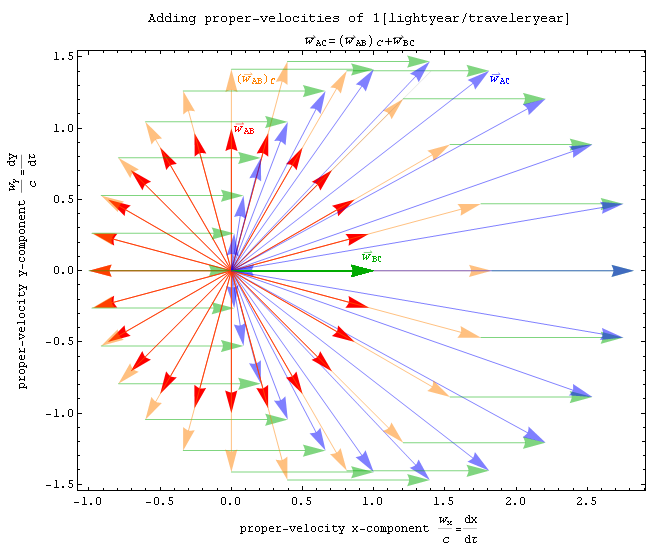# Expected Distance of Unit Vectors

Calculus Level 2Two unit vectors in two-dimensional space $\hat{\textbf{a}}$ and $\hat{\textbf{b}}$ are added together. The expected magnitude of the resulting vector $\textbf{a+b}$ is equal to $E.$ What is $\lfloor100E\rfloor?$

Also try Daniel Liu's Expected Distance on a Circle.

×